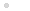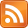### My CPU

Homebrew CPU construction / házi készítésű processzor

### Feedek## On (in)Efficiency of FunCPU

### 2014.08.11. 21:45 Budapesti álmodozó

On Data and Program Representation

The computation (expression reduction) process is based on string manipulations (copy, insert, replace, etc.). The input string is scanned symbol by symbol until the string is reduced to an integer. This may happen in some cases, but may not in others. In the course of this scanning/copying operations built-in functions as well user-functions are evaluated. We will refer to a series of such scan/copy activities as cycle, until the expression end tag is reached. Please note that there is some optimization at work, so that, for instance, when copying the string

FC FE FC 00 (representing inc dec inc 00)

it is immediately reduced (that is to say in the very same cycle during scan/copy loop) to

FC 00 (representing inc 00), and then to 01

In this way some cycles can be saved. Otherwise the chain of reduction would be like this:

FC FE FC 00 => FC FE 01 => FC 00 => 01

Note each reduction happens in a separate cycle.

Still the clear disadvantage of this evaluation process lies in the facts that:

• high number of cycles (this is further detailed in the section below) are required;
• a relatively big number of symbols are copied/moved in each cycle.

On CPU Cycles

The FunCPU is quite inefficient, that is to say, each calculation requires quite many cycles. Actually too many. This is basically due to the very low level built-in functions, i.e. inc and dec. To illustrate this, just imagine how two integers are added. For example, x+y requires just y cycles to increment x (using the definition of “add” function as outlined below), that is, to add y to x, not to mention the additional cycles required for unfolding the “add” function, evaluating the if-then-else expression, etc.

It is worth noting that although addition is commutative, the computation of x+y and y+x requires different number of cycles (and thus execution time) provided that x and y are different integers. Of course, to eliminate this undesired property, one can define a more clever addition function, which swaps its arguments initially in order to minimize the number of cycles required.

It is quite straightforward to think of introducing higher level functions, such as addition, subtraction, multiplication, division, etc. Needless to say, that with such richer set of built-in function the efficiency of computation process is highly increased at the price of a more complex architecture.

### Címkék: efficiencyfunctional programmingFunCPUreduction processexpression evaluation